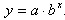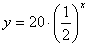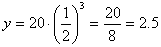Application
Example 1 Continued

Step 3. Write a model for the exponential decay.

Remember that the general formula for an exponential function isa is the initial value, so a = 20.
b
is the common ratio, so b = 1/2.

Therefore, the equation isStep 4. Determine the amount of radioactivity left after three weeks.

Since x represents the number of weeks, let x = 3.